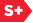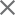### Proceedings Paper

New 2D discrete Fourier transforms in image processing
Format Member Price Non-Member Price
PDF \$17.00 \$21.00

Paper Abstract

In this paper, the concept of the two-dimensional discrete Fourier transformation (2-D DFT) is defined in the general case, when the form of relation between the spatial-points (x, y) and frequency-points (ω1, ω2) is defined in the exponential kernel of the transformation by a nonlinear form L(x, y; ω1, ω2). The traditional concept of the 2-D DFT uses the Diaphanous form 1 +yω2 and this 2-D DFT is the particular case of the Fourier transform described by the form L(x, y; ω1, ω2). Properties of the general 2-D discrete Fourier transform are described and examples are given. The special case of the N × N-point 2-D Fourier transforms, when N = 2r, r > 1, is analyzed and effective representation of these transforms is proposed. The proposed concept of nonlinear forms can be also applied for other transformations such as Hartley, Hadamard, and cosine transformations.

Paper Details

Date Published: 16 March 2015
PDF: 12 pages
Proc. SPIE 9399, Image Processing: Algorithms and Systems XIII, 939910 (16 March 2015); doi: 10.1117/12.2083389
Show Author Affiliations
Artyom M. Grigoryan, The Univ. of Texas at San Antonio (United States)
Sos S. Agaian, The Univ. of Texas at San Antonio (United States)

Published in SPIE Proceedings Vol. 9399:
Image Processing: Algorithms and Systems XIII
Karen O. Egiazarian; Sos S. Agaian; Atanas P. Gotchev, Editor(s)